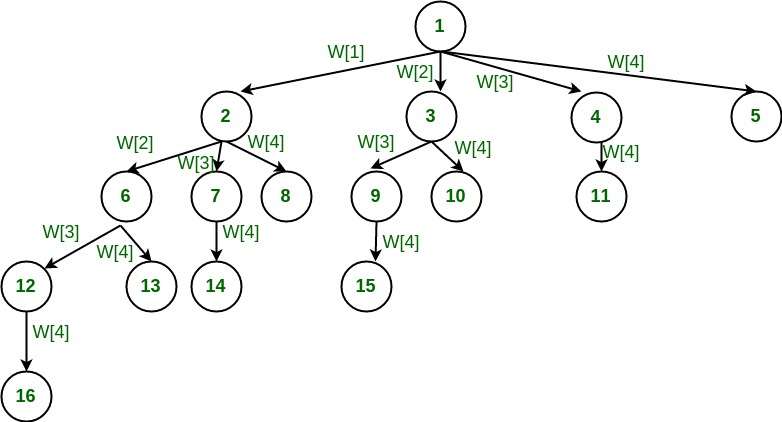# Find all the subsets whose sum is equal to 'K'

Challenging task: Given a linear array of 'n' elements, find the number of individual subsets of the array that can be formed in such a way that the sum of the subset is equal to an user-defined value 'K'.

Solution:   The simplest solution for this problem is to consider all possible subsets and find their sum. Count the number of subsets that add upto a sum value of 'K' and output that as the answer. Here, a total of 2^n combinations could be possible if elements are not repeated in the given array. Testing results for all 2^n combinations could be a cumbersome task. Hence, we use backtracking algorithm to produce faster results.

Backtracking Algorithm for Subset Sum

The  tree diagram below depicts approach of generating variable sized tuple.In the above diagramatic representation we can see that subsets are formed by forming a tree with multiple tuples. Now each node in the tree forms various subsets with its children. The sum value is recursively tested if their sum adds up to a value of 'K'. If the sum exceeds the value of 'K' the tree is discarded on that value and is tested similarly on other branches of the same tree.

# Following is C implementation of subset sum:

```#include <stdio.h>
#include <stdlib.h>

#define ARRAYSIZE(a) (sizeof(a))/(sizeof(a))

static int total_nodes;
// prints subset found
void printSubset(int A[], int size)
{
for(int i = 0; i < size; i++)
{
printf("%*d", 5, A[i]);
}

printf("n");
}

// inputs
// s            - set vector
// t            - tuplet vector
// s_size       - set size
// t_size       - tuplet size so far
// sum          - sum so far
// ite          - nodes count
// target_sum   - sum to be found
void subset_sum(int s[], int t[],
int s_size, int t_size,
int sum, int ite,
int const target_sum)
{
total_nodes++;
if( target_sum == sum )
{
// We found subset
printSubset(t, t_size);
// Exclude previously added item and consider next candidate
subset_sum(s, t, s_size, t_size-1, sum - s[ite], ite + 1, target_sum);
return;
}
else
{
// generate nodes along the breadth
for( int i = ite; i < s_size; i++ )
{
t[t_size] = s[i];
// consider next level node (along depth)
subset_sum(s, t, s_size, t_size + 1, sum + s[i], i + 1, target_sum);
}
}
}

// Wrapper to print subsets that sum to target_sum
// input is weights vector and target_sum
void generateSubsets(int s[], int size, int target_sum)
{
int *tuplet_vector = (int *)malloc(size * sizeof(int));

subset_sum(s, tuplet_vector, size, 0, 0, 0, target_sum);

free(tuplet_vector);
}

int main()
{
int weights[] = {10, 7, 5, 18, 12, 20, 15};
int size = ARRAYSIZE(weights);

generateSubsets(weights, size, 35);
printf("Nodes generated %dn", total_nodes);
return 0;
}
```

```Optimised C implementation to find the sum of subsets:#include <stdio.h>
#include <stdlib.h>

#define ARRAYSIZE(a) (sizeof(a))/(sizeof(a))

static int total_nodes;

// prints subset found
void printSubset(int A[], int size)
{
for(int i = 0; i < size; i++)
{
printf("%*d", 5, A[i]);
}

printf("n");
}

// qsort compare function
int comparator(const void *pLhs, const void *pRhs)
{
int *lhs = (int *)pLhs;
int *rhs = (int *)pRhs;

return *lhs > *rhs;
}

// inputs
// s            - set vector
// t            - tuplet vector
// s_size       - set size
// t_size       - tuplet size so far
// sum          - sum so far
// ite          - nodes count
// target_sum   - sum to be found
void subset_sum(int s[], int t[],
int s_size, int t_size,
int sum, int ite,
int const target_sum)
{
total_nodes++;

if( target_sum == sum )
{
// We found sum
printSubset(t, t_size);

// constraint check
if( ite + 1 < s_size && sum - s[ite] + s[ite+1] <= target_sum )
{
// Exclude previous added item and consider next candidate
subset_sum(s, t, s_size, t_size-1, sum - s[ite], ite + 1, target_sum);
}
return;
}
else
{
// constraint check
if( ite < s_size && sum + s[ite] <= target_sum )
{
// generate nodes along the breadth
for( int i = ite; i < s_size; i++ )
{
t[t_size] = s[i];

if( sum + s[i] <= target_sum )
{
// consider next level node (along depth)
subset_sum(s, t, s_size, t_size + 1, sum + s[i], i + 1, target_sum);
}
}
}
}
}

{
int *tuplet_vector = (int *)malloc(size * sizeof(int));

int total = 0;

// sort the set
qsort(s, size, sizeof(int), &comparator);

for( int i = 0; i < size; i++ )
{
total += s[i];
}

if( s <= target_sum && total >= target_sum )
{

subset_sum(s, tuplet_vector, size, 0, 0, 0, target_sum);

}

free(tuplet_vector);
}

int main()
{
int weights[] = {15, 22, 14, 26, 32, 9, 16, 8};
int target = 53;

int size = ARRAYSIZE(weights);

generateSubsets(weights, size, target);

printf("Nodes generated %dn", total_nodes);

return 0;
}
```

POST A NEW COMMENT

• Input (stdin)

Output (stdout)

Input (stdin)

Expected Output

Compiler Message

Input (stdin)

`2    3`

`5`
`5`
`5`